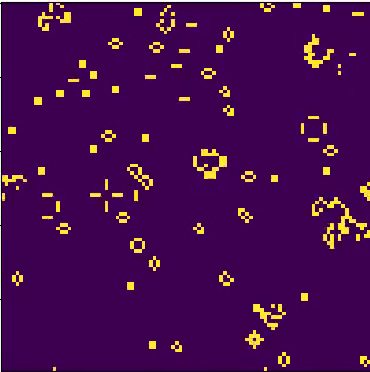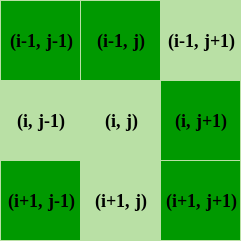Open in App
Not now

# Conway’s Game Of Life (Python Implementation)

• Difficulty Level : Medium
• Last Updated : 21 Jul, 2022

Conways’s Game Of Life is a Cellular Automation Method created by John Conway. This game was created with Biology in mind but has been applied in various fields such as Graphics, terrain generation,etc..The “game” is a zero-player game, meaning that its evolution is determined by its initial state, requiring no further input. One interacts with the Game of Life by creating an initial configuration and observing how it evolves, or, for advanced “players”, by creating patterns with particular properties.
How the game works
Because the Game of Life is built on a grid of nine squares, every cell has eight neighboring cells,as shown in the given figure. A given cell (i, j) in the simulation is accessed on a grid [i][j], where i and j are the row and column indices, respectively. The value of a given cell at a given instant of time depends on the state of its neighbors at the previous time step. Conway’s Game of Life has four rules.

1. If a cell is ON and has fewer than two neighbors that are ON, it turns OFF
2. If a cell is ON and has either two or three neighbors that are ON, it remains ON.
3. If a cell is ON and has more than three neighbors that are ON, it turns OFF.
4. If a cell is OFF and has exactly three neighbors that are ON, it turns ON.So since we know how it works, the next thing we need to figure it out that how to make it work.

Approach

1. Initialize the cells in the grid.
2. At each time step in the simulation, for each cell (i, j) in the grid, do the following:
1.  Update the value of cell (i, j) based on its neighbors, taking into account the boundary conditions.
2.  Update the display of grid values.

After we done here lets get our hands on code.

Requirements

1. numpy
2. matplotlib
3. argparse
4. pygame

Implementation: Now lets get it started

## Python3

 `# Python code to implement Conway's Game Of Life``import` `argparse``import` `numpy as np``import` `matplotlib.pyplot as plt``import` `matplotlib.animation as animation` `# setting up the values for the grid``ON ``=` `255``OFF ``=` `0``vals ``=` `[ON, OFF]` `def` `randomGrid(N):` `    ``"""returns a grid of NxN random values"""``    ``return` `np.random.choice(vals, N``*``N, p``=``[``0.2``, ``0.8``]).reshape(N, N)` `def` `addGlider(i, j, grid):` `    ``"""adds a glider with top left cell at (i, j)"""``    ``glider ``=` `np.array([[``0``,    ``0``, ``255``],``                       ``[``255``,  ``0``, ``255``],``                       ``[``0``,  ``255``, ``255``]])``    ``grid[i:i``+``3``, j:j``+``3``] ``=` `glider` `def` `addGosperGliderGun(i, j, grid):` `    ``"""adds a Gosper Glider Gun with top left``       ``cell at (i, j)"""``    ``gun ``=` `np.zeros(``11``*``38``).reshape(``11``, ``38``)` `    ``gun[``5``][``1``] ``=` `gun[``5``][``2``] ``=` `255``    ``gun[``6``][``1``] ``=` `gun[``6``][``2``] ``=` `255` `    ``gun[``3``][``13``] ``=` `gun[``3``][``14``] ``=` `255``    ``gun[``4``][``12``] ``=` `gun[``4``][``16``] ``=` `255``    ``gun[``5``][``11``] ``=` `gun[``5``][``17``] ``=` `255``    ``gun[``6``][``11``] ``=` `gun[``6``][``15``] ``=` `gun[``6``][``17``] ``=` `gun[``6``][``18``] ``=` `255``    ``gun[``7``][``11``] ``=` `gun[``7``][``17``] ``=` `255``    ``gun[``8``][``12``] ``=` `gun[``8``][``16``] ``=` `255``    ``gun[``9``][``13``] ``=` `gun[``9``][``14``] ``=` `255` `    ``gun[``1``][``25``] ``=` `255``    ``gun[``2``][``23``] ``=` `gun[``2``][``25``] ``=` `255``    ``gun[``3``][``21``] ``=` `gun[``3``][``22``] ``=` `255``    ``gun[``4``][``21``] ``=` `gun[``4``][``22``] ``=` `255``    ``gun[``5``][``21``] ``=` `gun[``5``][``22``] ``=` `255``    ``gun[``6``][``23``] ``=` `gun[``6``][``25``] ``=` `255``    ``gun[``7``][``25``] ``=` `255` `    ``gun[``3``][``35``] ``=` `gun[``3``][``36``] ``=` `255``    ``gun[``4``][``35``] ``=` `gun[``4``][``36``] ``=` `255` `    ``grid[i:i``+``11``, j:j``+``38``] ``=` `gun` `def` `update(frameNum, img, grid, N):` `    ``# copy grid since we require 8 neighbors``    ``# for calculation and we go line by line``    ``newGrid ``=` `grid.copy()``    ``for` `i ``in` `range``(N):``        ``for` `j ``in` `range``(N):` `            ``# compute 8-neighbor sum``            ``# using toroidal boundary conditions - x and y wrap around``            ``# so that the simulation takes place on a toroidal surface.``            ``total ``=` `int``((grid[i, (j``-``1``)``%``N] ``+` `grid[i, (j``+``1``)``%``N] ``+``                         ``grid[(i``-``1``)``%``N, j] ``+` `grid[(i``+``1``)``%``N, j] ``+``                         ``grid[(i``-``1``)``%``N, (j``-``1``)``%``N] ``+` `grid[(i``-``1``)``%``N, (j``+``1``)``%``N] ``+``                         ``grid[(i``+``1``)``%``N, (j``-``1``)``%``N] ``+` `grid[(i``+``1``)``%``N, (j``+``1``)``%``N])``/``255``)` `            ``# apply Conway's rules``            ``if` `grid[i, j]  ``=``=` `ON:``                ``if` `(total < ``2``) ``or` `(total > ``3``):``                    ``newGrid[i, j] ``=` `OFF``            ``else``:``                ``if` `total ``=``=` `3``:``                    ``newGrid[i, j] ``=` `ON` `    ``# update data``    ``img.set_data(newGrid)``    ``grid[:] ``=` `newGrid[:]``    ``return` `img,` `# main() function``def` `main():` `    ``# Command line args are in sys.argv, sys.argv ..``    ``# sys.argv is the script name itself and can be ignored``    ``# parse arguments``    ``parser ``=` `argparse.ArgumentParser(description``=``"Runs Conway's Game of Life simulation."``)` `    ``# add arguments``    ``parser.add_argument(``'--grid-size'``, dest``=``'N'``, required``=``False``)``    ``parser.add_argument(``'--mov-file'``, dest``=``'movfile'``, required``=``False``)``    ``parser.add_argument(``'--interval'``, dest``=``'interval'``, required``=``False``)``    ``parser.add_argument(``'--glider'``, action``=``'store_true'``, required``=``False``)``    ``parser.add_argument(``'--gosper'``, action``=``'store_true'``, required``=``False``)``    ``args ``=` `parser.parse_args()``    ` `    ``# set grid size``    ``N ``=` `100``    ``if` `args.N ``and` `int``(args.N) > ``8``:``        ``N ``=` `int``(args.N)``        ` `    ``# set animation update interval``    ``updateInterval ``=` `50``    ``if` `args.interval:``        ``updateInterval ``=` `int``(args.interval)` `    ``# declare grid``    ``grid ``=` `np.array([])` `    ``# check if "glider" demo flag is specified``    ``if` `args.glider:``        ``grid ``=` `np.zeros(N``*``N).reshape(N, N)``        ``addGlider(``1``, ``1``, grid)``    ``elif` `args.gosper:``        ``grid ``=` `np.zeros(N``*``N).reshape(N, N)``        ``addGosperGliderGun(``10``, ``10``, grid)` `    ``else``:   ``# populate grid with random on/off -``            ``# more off than on``        ``grid ``=` `randomGrid(N)` `    ``# set up animation``    ``fig, ax ``=` `plt.subplots()``    ``img ``=` `ax.imshow(grid, interpolation``=``'nearest'``)``    ``ani ``=` `animation.FuncAnimation(fig, update, fargs``=``(img, grid, N, ),``                                  ``frames ``=` `10``,``                                  ``interval``=``updateInterval,``                                  ``save_count``=``50``)` `    ``# # of frames?``    ``# set output file``    ``if` `args.movfile:``        ``ani.save(args.movfile, fps``=``30``, extra_args``=``[``'-vcodec'``, ``'libx264'``])` `    ``plt.show()` `# call main``if` `__name__ ``=``=` `'__main__'``:``    ``main()`

Without passing any command line arguments.

Now lets turn up things a little, let’s see what happens if add updates the animation every 500 milliseconds and setting up the dimensions 32X32 and also using the initial glider pattern.

`python 'filename.py' --grid-size 32 --interval 500 --glide`

You can try manipulating this code to create different simulation using this.